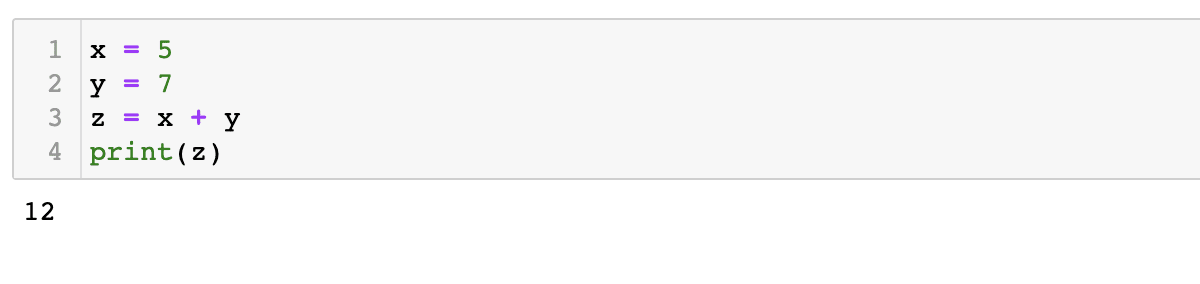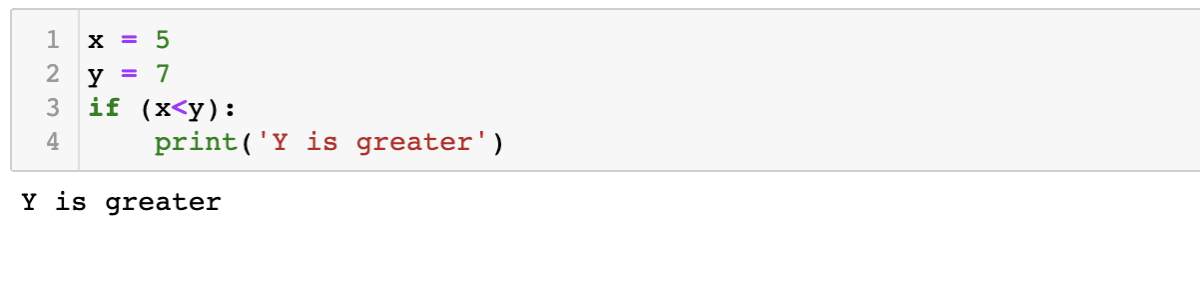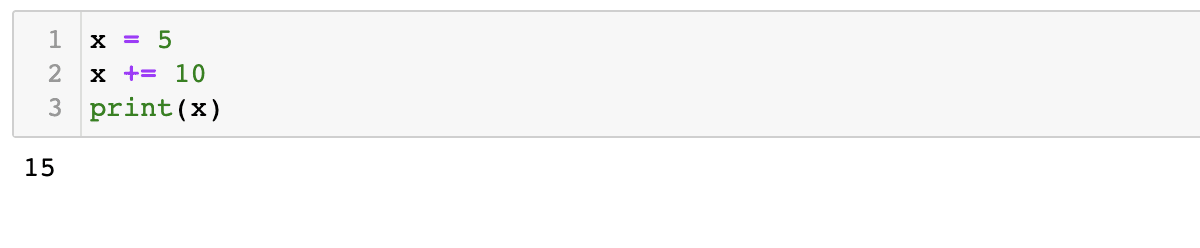Skip to content

# Operators

Operators are symbols that perform operations on variables and values. Based on the class of operator used, they can be adapted towards different applications.

## Arithmetic Operators:

Arithmetic operators are used to perform simple arithmetic operations.

 Operator Function Example `+` addition 5+2 =7 `-` subtraction 5-2 =3 `*` multiplication 5*2 =10 `/` division 5/2 =2.5 `%` computes remainder 5%2 =1 `//` floor division (will not return floating point numbers 5//2= 2
```x = 5
y = 7
z = x + y
print(z)```## Comparison Operators:

Comparison operators are used to check conditions and compare two numbers or variables.

 Operator Function Example `==` Checks if two numbers are equal x == y `!=` checks if two numbers are not equal x != y `<` checks whether a number is less than another x < y `>` checks whether a number is greater than another x > y `<=` checks whether a number is less than or equal to another x <= y `>=` checks whether a number is greater than or equal to another x >= y
```x = 5
y = 7
if (x<y):
print('Y is greater')```## Assignment Operators:

Assignment operators are used to assign a value to a variable.

 Operator Example Function = a=8 a is assigned the value of 8 += a+=8 this is useful in case of an iterative operation; similar functions can be defined for other arithmetic operators
```x = 5
x += 10
print(x)```